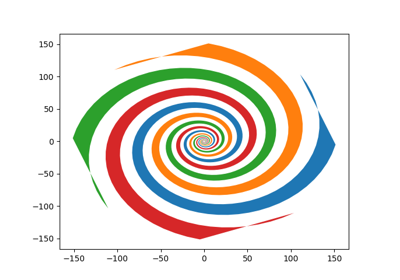# matplotlib.pyplot.fill¶

matplotlib.pyplot.fill(*args, data=None, **kwargs)[source]

Plot filled polygons.

Parameters: args : sequence of x, y, [color] Each polygon is defined by the lists of x and y positions of its nodes, optionally followed by a color specifier. See matplotlib.colors for supported color specifiers. The standard color cycle is used for polygons without a color specifier. You can plot multiple polygons by providing multiple x, y, [color] groups. For example, each of the following is legal: ax.fill(x, y) # a polygon with default color ax.fill(x, y, "b") # a blue polygon ax.fill(x, y, x2, y2) # two polygons ax.fill(x, y, "b", x2, y2, "r") # a blue and a red polygon  a list of :class:~matplotlib.patches.Polygon **kwargs : Polygon properties

Notes

Use fill_between() if you would like to fill the region between two curves.

Note

In addition to the above described arguments, this function can take a data keyword argument. If such a data argument is given, the following arguments are replaced by data[<arg>]:

• All arguments with the following names: 'x', 'y'.

Objects passed as data must support item access (data[<arg>]) and membership test (<arg> in data).

## Examples using matplotlib.pyplot.fill¶Interactive functionsFill Spiral